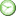# Middle and Upper Levels ISEE Math Practice Test 31

### Test Information10 questions10 minutes

Take more free ISEE Mathematics Achievement Practice Tests available from crackssat.com.

1. How many numbers between 1 and 100, inclusive, are both prime and a multiple of 4 ?

• A. 0
• B. 12
• C. 20
• D. 25

2. How many factors do the integers 24 and 81 have in common?

• A. 1
• B. 2
• C. 3
• D. 4

3. If the final total of a dinner bill-after including a 25% tip-is \$50, what was the cost of the dinner before including the tip?

• A. \$12.50
• B. \$25.00
• C. \$37.50
• D. \$40.00

4. How many numbers between 10 and 150 inclusive are multiples of both 3 and 5 ?

• A. 9
• B. 10
• C. 15
• D. 20

5. 34 × 34 × 34 =

• A. 38
• B. 312
• C. 364
• D. 3(34)

6. For what integer value of x does x4 = 4x + 8 ?

• A. 1
• B. 2
• C. 3
• D. 4

7. If 18 = 3(3x - 6), then x + 6 =

• A. 4
• B. 6
• C. 10
• D. 26

8. What is the largest multiple of 6 that is less than 53 ?

• A. 36
• B. 48
• C. 52
• D. 54

9. One-third of the cars available for purchase at a used car dealership are silver. If there are 24 silver cars at the dealership, how many used cars are available for purchase?

• A. 8
• B. 24
• C. 48
• D. 72

10. Marie's garden contains 30 yellow roses, 50 red roses, and 40 white roses. Of the roses in her garden, what percent are NOT yellow?

• A. 30%
• B. 50%
• C. 75%
• D. 90%